An Accelerator-based Logistic Map Image Cryptosystems for Grayscale Images

M. Raviraja Holla1,*, Alwyn R. Pais2 and D. Suma3

1Department of Information and Communication Technology, Manipal Institute of Technology, Manipal Academy of Higher Education (MAHE), Manipal,

Karnataka, India

2Information Security Research Lab., Department of Computer Science and Engineering, National Institute of Technology Karnataka, Surathkal, India

3Department of Computer Science and Engineering, Manipal Institute of Technology, Manipal Academy of Higher Education (MAHE), Manipal,

Karnataka, India

E-mail: raviraj.holla@manipal.edu

*Corresponding Author

Received 22 October 2020; Accepted 31 January 2021; Publication 13 May 2021

## Abstract

The logistic map is a class of chaotic maps. It is still in use in image cryptography. The logistic map cryptosystem has two stages, namely permutation, and diffusion. These two stages being computationally intensive, the permutation relocates the pixels, whereas the diffusion rescales them. The research on refining the logistic map is progressing to make the encryption more secure. Now there is a need to improve its efficiency to enable such models to fit for high-speed applications. The new invention of accelerators offers efficiency. But the inherent data dependencies hinder the use of accelerators. This paper discusses the novelty of identifying independent data-parallel tasks in a logistic map, handing them over to the accelerators, and improving their efficiency. Among the two accelerator models proposed, the first one achieves peak efficiency using coalesced memory access. The other cryptosystem further improves performance at the cost of more execution resources. In this investigation, it is noteworthy that the parallelly accelerated logistic map achieved a significant speedup to the larger grayscale image used. The objective security estimates proved that the two stages of the proposed systems progressively ensure security.

Keywords: Accelerator, logistic map, encryption, cryptography.

## 1 Introduction

A successful attack on an Information Technology (IT) system is called a security incident. Security incidents have drastically increased over the years in the world of the Internet. Due to the open unsafe communication channel and the broad clients of the Internet, it is easy for an adversary to exploit vulnerabilities and commit security incidents. Dynamically preventive security is only a viable solution than any detective or recovery security possibilities. The emerging image cryptography models provide such security solutions.

The invention of image encryption by scrambling the image dates back to 1975 . The original image is converted to an unrecognizable image using a photographic approach. This reversible and reproducible transformation used a code plate comprising of unique random binary codes. A reversible logical operation decides any bit of the scrambled image on the corresponding pair of bits in the secret image and the code plate. The users have the code plates authorized to decipher the plain-image. The original image, when closely contacted with the code plate revealed the secrecy. The researchers in  used a chaotic map to encrypt the image. The pixel correlation in the two-dimensional representation of an image is high. So, authors in  used a high-dimensional map to de-correlate this correspondence by transposition of the pixels. This operation is called a permutation. Another procedure called diffusion makes the encrypted image distinctly different from the plain-image. This logistic map is a chaotic map of polynomial recurrence relation of degree 2. This function is a low-dimensional relation that can be easily implemented for an encryption . In , the researchers analyzed the two primary operations based on a logistic map for evaluation. These operations are permutation and diffusion. The investigators interchanged the sequencing of these two operations and evaluated the effectiveness. This evaluation can be considered a reference model for selecting the effective and efficient process and the ordering of activities for chaotic map encryption.

Other than the pixel correlation in two-dimensional space, images have characteristics of high-capacity and redundancy of computations. Due to these two features, the logistic map based encryption is not affordable for on-line communications and real-time applications . The last decade has witnessed an exponential growth in parallel processing power and paradigms . An efficient logistic map permutation-diffusion-based image cryptosystems are proposed in this paper. These systems exploit accelerators to improve the elapsed time spent on processing. The proposed cryptosystems are objectively analyzed using various metrics to prove that the system is robustly secured.

## 2 Background and Motivation

The use of chaotic maps in the image cryptography is growing. Recently, the application of the Chaotic Map for the encryption is exemplified in  using the DNA encoding technique. The chaos is the base for the key-image. The key and secret images are encoded row by row with DNA rules. The logistic map ensures that different rows are encoded – the final cipher image obtained by repeating the operations column by column. Table 1 consolidates and compares the literature discussed so far.

Table 1 Comparison analysis of existing literature

 Author Features Pros and Cons Hines  Original image transformed to a scrambled image and later restored using same code plates. Need reduction in computational cost. Chen et al.  Used three-dimensional cat chaotic map for transposition of pixels of the original image and another map to de-correlate pixels of the original and cipher-images. Improved security. Speedy encryption. Liu et al.  Variable parameter one-dimensional map used for pixel shuffling and dynamical reversible algorithm used for the encryption. High security. Reduced execution time for encryption. But do not fit for real-time applications. Wang et al.  Analysed effect of the permutation and diffusion operations based on the chosen chaotic maps on security. Concluded that effect of the order of permutation and diffusion on security is specific to the chosen. He et al.  Proposed parallel two-dimensional Arnold chaotic map for pixel shuffling and three-dimensional Liu map system for the encryption. An efficient model that can be applied to real-time applications. Wang and Liu  The parameters needed for the encryption algorithm are derived from Piecewise Linear Map and Logistic Map. DNA rule is used for encoding. It is a serial model. But the model is computationally intensive. So, the researchers reserved their future work making this model efficient using an accelerator.

The authors in  identified a plain-text attack vulnerability in a specific chaotic mapping cryptography algorithm. The researchers then introduced a chaos-based image replacement to withstand plain-text attacks. This novel method retained other qualities of the technique they analyzed. This article lists features that may be required by any chaotic cryptosystem. The most important of them is the flexibility of selecting any chaotic mapping, vast chaotic space, reduced redundancies or repetitions, secret key sensitivity, good security, and proper pixel distribution after encryption.

Recently, concerns about chaotic cryptosystem efficiency have begun to emerge. Here are some of the findings that inspire adding efficiency to the cryptosystem list of desired features. These findings also provide both direct and indirect reasons for that proposition. If the cryptosystem’s emerging applications are the immediate causes, its features are indirect causes. Real-time [5, 9], high-protective [5, 10, 11], remote sensing , computer vision , deep learning , super-resolution [15, 16], and multimedia  applications are important indirect causes. Transmission cost , hardware cost, and the power consumption [19, 20], is an example of an indirect cause. The use of multi-type maps , the size of the images, and some redundancy  can also help with efficiency through parallelism.

Nonetheless, all these inventions used a modern parallel paradigm. Parallel computing has revolutionized the field of computing [22, 23]. The field cryptography has to gain a lot from concurrency. The article  overviews the parallel-paradigm development overt the time-line. Notably, there is a growing demand for parallel thinking for better efficiency. The main part of any structured cryptography included chaos-based cryptography . However, image cryptography algorithms based on complex, chaotic maps or other designs cannot be easily parallelized . These opportunities and the challenges have driven the efficiency of the logistic-map cryptography with state of the art parallel technology. This paper describes the investigation of such a unique and novel accelerated logistic map cryptosystems.

## 3 Method

### 3.1 Accelerated Logistic Map Image Cryptosystems

The architecture in the proposed systems use two steps proposed in  during encryption. They are the permutation and diffusion. Decryption reversely reuses these two steps. The permutation – diffusion model which uses logistic map is shown in Figure 2. Equation (1) denotes the logistic map equation used in this model.

 $xn=r⁢xn⁢(1-xn)$ (1)

The $r$ in Equation (1) is a positive constant. This quadratic map has a very complex behavior in various iterations, as shown in Figure 1 for the value of $r=4$.Figure 1 Logistic function (red) and its behaviour in various iterations .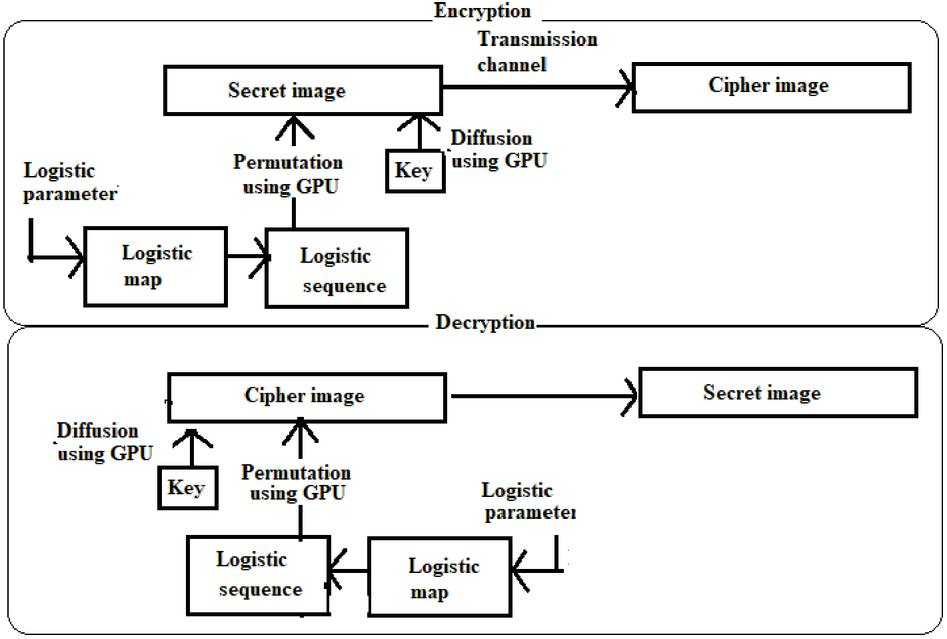Figure 2 Permutation – diffusion logistic map architecture.

In the proposed systems, the initial values set to $r=3.94$ and $x0=0.4$. Equation (1) generates the logistic sequence of double-precision numbers. This sequence is placed in sequential order. The pixel position in each row is changed based on this sequence. The diffusion generates the encrypted image by performing a bitwise XOR operation on every pixel with a variable say $l$, where $l$ is obtained by multiplying each element of the generated sequence with a key 987654321012345 and performing mod 255. The decryption reverses these two steps to produce the original image. Initially, we develop the CPU-based logistic map architecture as a basis to propose two efficient accelerator-based cryptosystems. In this accelerator-based category, Figure 5 shows the first version, which is a refined model shown in Figure 3. The similarity among these two models is that both these models generate threads equal to the total image height. However, in the naive model (Figure 3), neighbouring threads store the interleaved memory addresses in each iteration.

The consequence is two-fold. The interleaved references for single access lead to memory underutilization because the data available between these references remain unused for that access. This misaligned and non-coalesced access often leads to multiple memory accesses. Instead of directly processing, processing the transpose of the image guarantees coalesced memory access. By transposing the image in Figure 5, adjacent threads generate adjacent addresses. As there are now regular access patterns unlike interleaved, many accesses otherwise are coalesced to one, improving memory bandwidth utilization to achieve peak performance . Figure 4 shows the coalesced and non-coalesced accesses.

In the second accelerator version, instead of image height, threads equal to the image size are created as in Figure 6. This model improves speedup further by eliminating the thread traversals.Figure 3 Thread organization and pixel mapping in general accelerator model.Figure 4 Global memory access  (a) Aligned and successive access (b) Mis-aligned access.Figure 5 Thread organization and pixel mapping in accelerator based version 1.Figure 6 Thread organization and pixel mapping in accelerator based version 2.

Algorithm 1 Manages both CPU and GPU resources. Also, launches the kernels $e⁢n⁢c⁢r⁢y⁢p⁢t⁢i⁢o⁢n⁢_⁢c⁢o⁢a⁢l⁢e⁢s⁢c⁢e⁢d⁢()$ and $e⁢n⁢c⁢r⁢y⁢p⁢t⁢i⁢o⁢n⁢_⁢t⁢h⁢r⁢e⁢a⁢d⁢_⁢p⁢e⁢r⁢_$ $p⁢i⁢x⁢e⁢l⁢()$.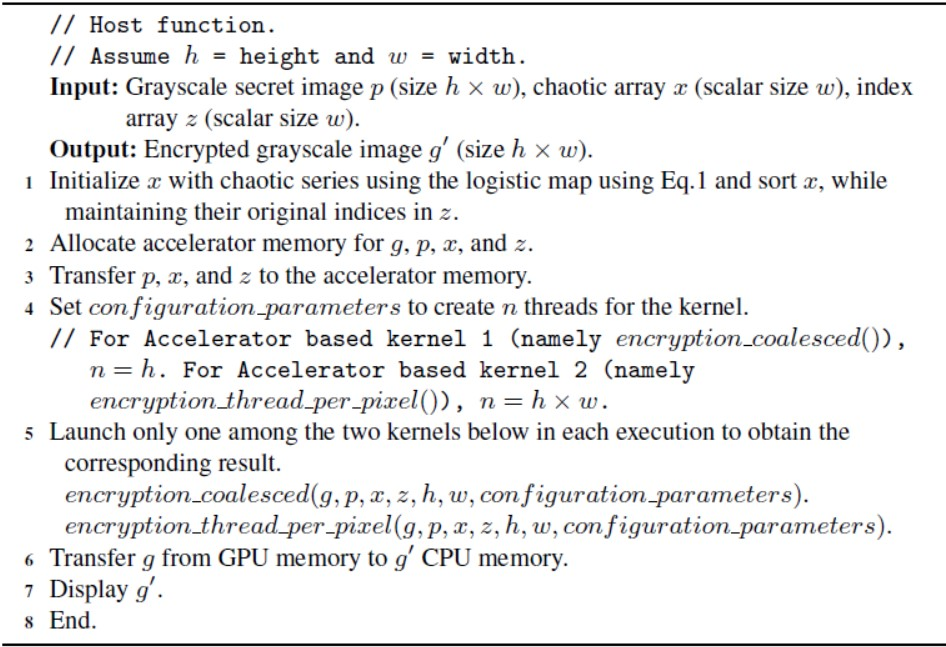Algorithm 2 $e⁢n⁢c⁢r⁢y⁢p⁢t⁢i⁢o⁢n⁢_⁢c⁢o⁢a⁢l⁢e⁢s⁢c⁢e⁢d⁢(g,p,x,z,h,w)$: Generates an encrypted image after permutation and diffusion.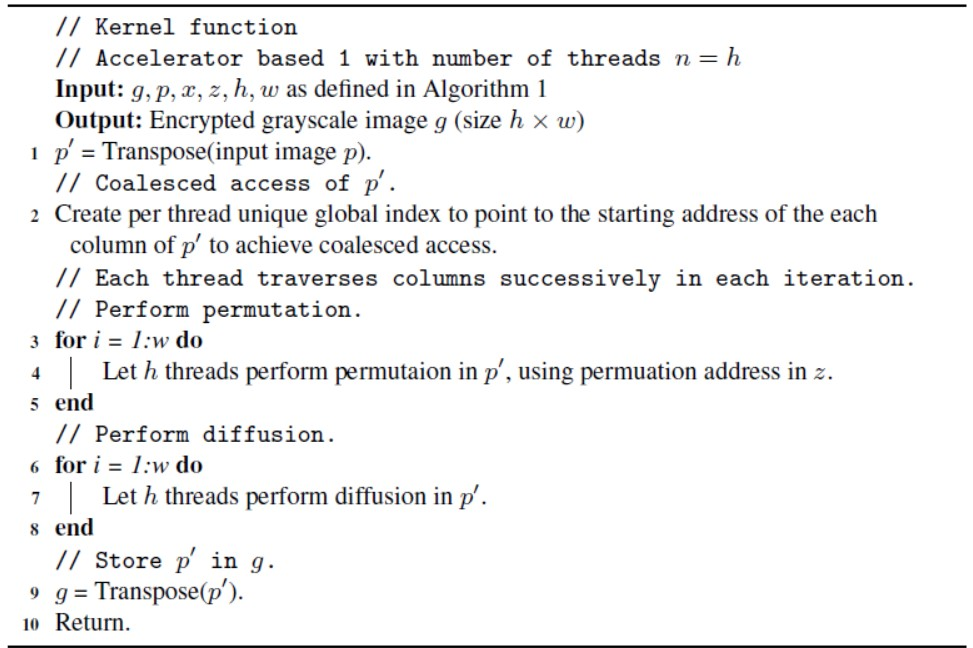Algorithm 3 $e⁢n⁢c⁢r⁢y⁢p⁢t⁢i⁢o⁢n⁢_⁢t⁢h⁢r⁢e⁢a⁢d⁢_⁢p⁢e⁢r⁢_⁢p⁢i⁢x⁢e⁢l⁢(g,p,x,z,h,w)$: Generates an encrypted image after permutation and diffusion.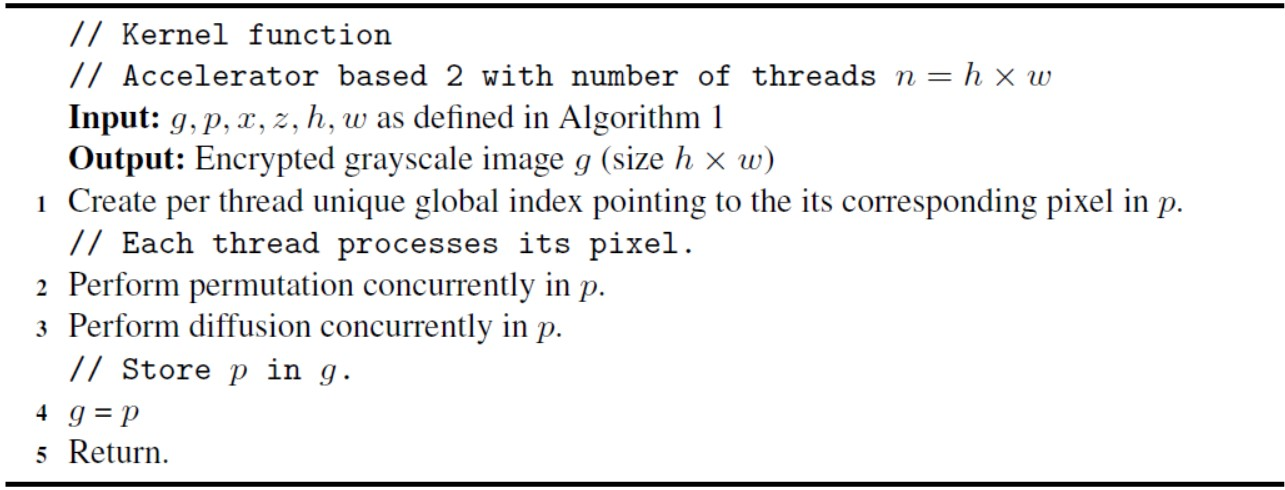### 3.2 Accelerator Programming Using PyCUDA

Algorithm 1, Algorithm 2, and Algorithm 3 show the host function, accelerator-based two versions of the proposed system, as explained in Section 3.1.

### 3.3 Result and the Speedup of the Proposed Systems

Table 2 shows the total execution times of permutation and diffusion of three versions explained in Section 3.1. The increase in image size significantly increases the execution time in the CPU based version. Both accelerator-based versions are almost neutral to image size. The run time rises slightly for the rise in the image’s width in the accelerator-based first version. The speedup is the efficiency of the accelerator-based models over the CPU-based model. The speedup of the first version of the accelerator is increased by 3347 times while the latter is about 20000 times, for the image size $1024×1024$. This speedup rises significantly as the image size increases. Figure 7 shows the speeds of the proposed system with its two variants. We also compared the execution times of the proposed models with the previous models in Table 3. The experimental values proved that our algorithms significantly outperform those models concerning the execution time enabling them eligible for real-time applications.

Table 2 The execution times (in seconds) for permutation and diffusion

 Environment Grayscale image size (Height x Width) $256×256$ $512×512$ $1024×1024$ CPU based 2.1024 3.3021 6.0254 Accelerator based 1 0.0016 0.0017 0.0018 Accelerator based 2 0.0002 0.0002 0.0003Figure 7 Speedup of the proposed systems during encryption.Figure 8 Image transformations during encryption (a) Secret image (b) Permuted image (c) Encrypted image.

For the grayscale image in Figure 8(a), Figure 8(b) and Figure 8(c) shows the transformation after permutation and diffusion during encryption, respectively. The decryption produces images in the reverse order.

### 3.4 Experimental Environment

PARAM Shavak Super Computing hardware with a CPU: Intel®Xeon ® – E5  – 2670 with two cores each containing 24 cores, 8TB RAM, 2.3 GHz. and single NVIDIA®Tesla K40 GPU: containing 2880 cores, 12 GB GDDR5, 745 MHz, PyCUDA to access NVIDIA’s Compute Unified Device Architecture (CUDA) parallel API from Python and OpenCV-Python.Figure 9 Histogram of (a) Secret image, (b) Permuted image, and (c) Encrypted image.

## 4 Objective Security Assessments of the Proposed Systems

Two general categories of assessing the quality of an intermediate or final image relative to a reference image are subjective and objective . The humans directly perceive and evaluate the image quality in the subjective-category. The objective-category uses computation to predict image ranking. However, subjective analysis is expensive in terms of time and budget. Also, the image computing can not make use of it for optimization . For cryptography, it is appropriate to consider security as an essential quality attribute. This section discusses a few objective image security assessments of the proposed systems.

### 4.1 Histogram Analysis

A histogram shows the number of pixels in the equal intervals between the inclusive minimum and maximum intensity values. Figure 9 shows the histograms of the secret, permuted, and encrypted images. Since the pixels in the permuted image are the relocated pixels of the secret image, its tone distribution is the same as the original image. So their histograms (Figure 9(b) and Figure 9(a)) are the same, respectively. But the histogram of the encrypted image (Figure 9(c)) is different as it changes the tone intensity of the permuted image. Also, the fact that the tone distribution is almost equal in the encrypted image authenticates how secure is the encrypted image.

### 4.2 Shannon Entropy Analysis

The information entropy ($H⁢(U)$) is a metric to quantify the randomness in an image. Equation (2) defines Shannon’s entropy , with $p⁢(uj)$ representing grayscale probability distribution. For 256 grayscale values of an image, $H⁢(U)$ lies in the range $(0≤H⁢(U)≤8)$. $H⁢(U)=0$ when the image pixels have a constant value. $H⁢(U)=8$ for the uniform pixel distribution in the histogram. From the table, it is evident that the $H⁢(U)$ of the encrypted image (Figure 8(c)) approches the maximum theoretic 8 . The computed entropy values of the secret (Figure 8(a)) and the permuted images (Figure 8(b)) are 7.446 and 7.459, respectively. Table 4 lists the entropy values of the secret image, permuted image, and the encrypted image. Figure 10 shows the Shannon entropy, $H⁢(U)$, analysis of the proposed system. Therefore the proposed cryptosystem is more secured.

 $H⁢(U)=∑j=0255p⁢(uj)⁢log2⁡p⁢(uj)$ (2)

Table 3 Comparison of objective security assessments of the proposed systems with the previous investigations

 Previous Investigations References (Ref.) Execution time (seconds) for image sizes 256, 512, and 1024. Entropy $H⁢(U)$ $S⁢S⁢I⁢M$ $M⁢S⁢E$ $P⁢S⁢N⁢R$ Pearson Correlation $rp⁢q$ $N⁢P⁢C⁢R$ $U⁢A⁢C⁢I$ $rh$ $rv$ $rd$ Ref.  0.3456 1.4016 5.3793 7.897 17.237 47.8 31.3 -0.0070 -0.0015 0.0054 98.71 31.9715 Ref.  0.4139 1.6877 6.8321 7,997 16.876 50.7 31.08 0.0061 0.0067 -0.0070 99.60 32.0100 Ref.  0.2854 1.1612 4.3270 7.997 19.761 40.3 32.07 -0.0042 0.0114 -0.0074 99.08 33.5612 Ref.  0.0933 0.3901 1.4803 7.999 15.947 7319 9.486 -0.0133 0.0007 0.0077 100 33.5515 Ref.  0.0629 0.2673 1.2157 7.993 12.116 1451 16.51 -0.0209 -0.0028 -0.0030 100 33.4183 Proposed Models CPU based 2.1024 3.3021 6.0254 7.998 9.018 8143 9.023 -0.0087 -0.0279 0.0246 100 33.4732 Accelerator based 1 0.0016 0.0017 0.0018 7.999 9.015 8141 9.023 0.0035 0.0037 -0.0095 100 33.4745 Accelerator based 2 0.0002 0.0002 0.0003 7.998 9.014 8143 9.023 0.0039 0.0035 -0.0098 100 33.4637

Table 4 Shannon entropy i.e., $H⁢(U)$ analysis

 Image $H⁢(U)$ Figure 8(a) 7.446 Figure 8(b) 7.459 Figure 8(c) 7.998

Table 3 reveals that the proposed models achieved the ideal entropy estimate when compared to the existing models.

### 4.3 Structural Similarity Index (SSIM) Analysis

The Structural Similarity Index $(-1≤S⁢S⁢I⁢M≤1)$ is a spatial domain metric that compares luminance, contrast, and structure of two images of a single capture [35, 28]. The $S⁢S⁢I⁢M=1$ for the two identical images and approaches minimum for the distinct images. The Equation (3) gives the $S⁢S⁢I⁢M$ for the comparison of a distorted image, $q$ with a reference plain image, $p$.

 $S⁢S⁢I⁢M⁢(p,q)=[l⁢(p,q)]α.[c⁢(p,q)]β.[s⁢(p,q)]γ$ (3)

Where,

 $l⁢(p,q)=2⁢μp⁢μq+C⁢1μp2+μq2+C⁢2c⁢(p,q)=2⁢σp⁢σq+C⁢2σp2+σq2+C⁢2s⁢(p,q)=σp⁢q+C⁢3σp⁢σq+C⁢3$

Equation (4) is the simplified form of Equation (3), assuming $α=β=γ=1$ and $C⁢3=C⁢2/2$.

 $S⁢S⁢I⁢M⁢(p,q)=(2⁢μp⁢μq+C⁢1)⁢(2⁢σp⁢q+C⁢2)(μp2+μq2+C⁢1)⁢(σp2+σq2+C⁢2)$ (4)

where $σp,σq$ are the local standard deviations of $p$ and $q$, $μp,μq,$ are the means of $p$ and $q$, and $σp⁢q$ is the cross-covariance of $p$ and $q$.

When it comes to security, the $S⁢S⁢I⁢M$’s low value indicates that the image $q$ is more different from image $p$ and provides more protection. Table 5 lists the $S⁢S⁢I⁢M$ values of the two compared images $p$ and $q$. In the first row, $p$ = Figure 8(a) (i.e., the original image) and $q$ = Figure 8(b) (i.e., the permuted image). Similarly, it is possible to interpret the second and third rows of this table. From the table, it is evident that the $S⁢S⁢I⁢M$ of the encrypted image ($q$ $=$ Figure 8(c)) is almost zero compared to the secret image ($p$ $=$ Figure 8(a)), indicating that the encrypted image is distinctly different from the secret image. It means that the encrypted image is least vulnerable to attacks and more secure. Figure 11 shows the $S⁢S⁢I⁢M$ analysis of the proposed system. We also demonstrate in Table 3 that our algorithms outperform other existing algorithms concerning SSIM.

Table 5 The $S⁢S⁢I⁢M$, $M⁢S⁢E$, and $P⁢S⁢N⁢R$ between two images in the proposed system

 Image $p$ Image $q$ $S⁢S⁢I⁢M$ $M⁢S⁢E$ $P⁢S⁢N⁢R$ Figure 8(a) Figure 8(b) 0.4463 4369 11.73 Figure 8(b) Figure 8(c) 0.4639 7783 9.219 Figure 8(a) Figure 8(c) 0.0098 8143 9.023Figure 10 Shannon entropy i.e., $H⁢(U)$ analysis.

### 4.4 Mean Square Error ($M⁢S⁢E$) Analysis

There are two standard error-based related image quality measures . They are the mean squared error ($M⁢S⁢E$) and the peak signal-to-noise ratio ($P⁢S⁢N⁢R$). Section 4.5 covers this relationship with a discussion on $P⁢S⁢N⁢R$. Let $h$ and $w$ represent the height and the width of the image. Let $p={p(i,j)|(0≥i≤h-1),(0≥j≤w-1)}$ and $q={q(i,j)|(0≥i≤h-1),(0≥j≤w-1)}$ represent the reference and the target images, respectively, where $p⁢(i,j)$ and $q⁢(i,j)$ represent the intensities of the pixels at $(i,j)$ in $p$ and $q$ respectively. $M⁢S⁢E$ is defined in Equation (5).Figure 11 $S⁢S⁢I⁢M$ index analysis.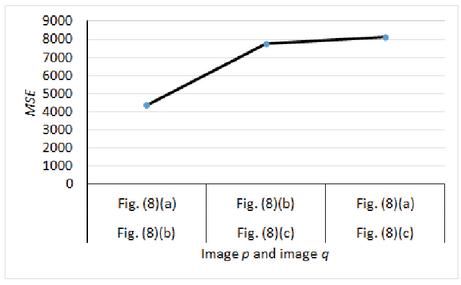Figure 12 $M⁢S⁢E$ analysis.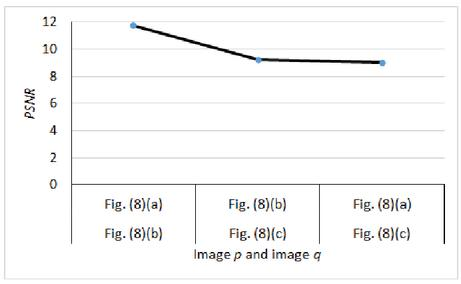Figure 13 $P⁢S⁢N⁢R$ analysis.

Higher the $M⁢S⁢E$, the better is the encryption meaning that the reference and the target images are dissimilar. Table 5 contains $M⁢S⁢E$ between any two pairs of the secret image, permuted image, and the encrypted image. Figure 12 shows the plot of these values. The increasing $M⁢S⁢E$ demonstrates that security increases as the image moves from permutation to the diffusion stage. Empirical values of MSE listed in Table 3 prove that the proposed cryptosystems achieved considerably high $M⁢S⁢E$ scores.

 $M⁢S⁢E⁢(p,q)=1h⁢w⁢∑i=0h-1∑j=0w-1[p⁢(i,j)-q⁢(i,j)]2$ (5)

### 4.5 Peak-signal to Noise Ratio (PSNR) Analysis

Root Mean Squared Error ($R⁢M⁢S⁢E$) of the two images, $p$ and $q$ is the square root of their $M⁢S⁢E$, as shown in Equation (6). Unlike $M⁢S⁢E$, it penalizes more the larger errors. $P⁢S⁢N⁢R$ is an approximation to the human visual system perception of the obtained image quality. Equation (7) defines $P⁢S⁢N⁢R$ using $R⁢M⁢S⁢E$. It is the ratio of the maximum pixel signal to the $R⁢M⁢S⁢E$. The maximum signal for the 8-bit depth grayscale image is 255. It is customary to express $P⁢S⁢N⁢R$ in the logarithmic decibel ($d⁢B$) scale.

Intuitive from the security perspective, the negatively-oriented $P⁢S⁢N⁢R$ score offers more security. Table 5 contains $P⁢S⁢N⁢R$ scores. Figure 13 shows the reduced $P⁢S⁢N⁢R$ scores during encryption in the proposed system.

 $R⁢M⁢S⁢E⁢(p,q)=M⁢S⁢E⁢(p,q)$ (6) $P⁢S⁢N⁢R⁢(p,q)=20.log10⁡255R⁢M⁢S⁢E⁢(d⁢B)$ (7)

When compared to $P⁢S⁢N⁢R$ of the previous works in Table 3, our models scored significantly reduced $P⁢S⁢N⁢R$ values.

### 4.6 Pearson Correlation

Equation (8) represents the Pearson correlation score $rp⁢q$ $(-1≤rp⁢q≤1)$ used to obtain horizontal, vertical, and diagonal pixel correlation scores $rh$, $rv$, and $rd$ of the encrypted image for $n$ random pixel samples.

 $rp⁢q=∑i=1n((pi-1n⁢∑j=1npj)×(qi-1n⁢∑j=1nqj))∑i=1n(pi-1n⁢∑j=1npj)2×∑i=1n(qi-1n⁢∑j=1nqj)2$ (8)

Where $p$ and $q$ are the intensity values of an adjacent pixel pair. Optimal encryption results in highly de-correlated neighboring pixels in the cipher image. Table 3 contains the optimal $rh$, $rv$, and $rd$ indices achieved in the proposed accelerator-based systems.

### 4.7 NPCR and UACI

The number of Pixels Change Rate ($N⁢P⁢C⁢R$) and Unified Average Changing Intensity ($U⁢A⁢C⁢I$) are the two estimations to evaluate the consequence of a bit change in the secret image on the encrypted image. A cryptosystem with $N⁢P⁢C⁢R$ and $U⁢A⁢C⁢I$ values exceeding 33.4635% and 99.6094% is not vulnerable to differential abuse . These two parameters are defined in Equations (9) and (10). Let $q1$ and $q2$ be the cipher images of the same secret image $p$ before and after a single bit change.

 $N⁢P⁢C⁢R=∑i,jq′⁢(i,j)h⁢w×100%$ (9)

In Equation (9), $q′⁢(i,j)=1$, if $q1⁢(i,j)≠q2⁢(i,j)$, otherwise $q′⁢(i,j)=0$.

 $U⁢A⁢C⁢I=1h⁢w⁢∑i,j|q1⁢(i,j)-q2⁢(i,j)|255×100%$ (10)

The Table 3 contains $N⁢P⁢C⁢R$ and $U⁢A⁢C⁢I$ values of the proposed accelerator-based models and the previous works. These values indicate that a one-bit change of the secret image changes all bits in the encrypted image.

## 5 Conclusion

Just as important as how much security image encryption provides, it must do so in real-time. Although the logistic map development to secure image is still underway, a new model to improve its efficiency is presented here. Permutation and diffusion are two main functions in the permutation-diffusion model logistic map encryption. This paper proposes a novel way of exploiting the data-parallel tasks in these two functions using an accelerator-the rest of the tasks assigned to the CPU because of their data dependency. Comparing the speeds obtained by creating two accelerator versions of the logistic map is made with the CPU-based logistic map. These models can be used for real-time applications because the speed achieved with the use of accelerators is enormous. The security metrics showed that the proposed system’s security is progressive as the secret image transforms through its stages. Besides, the performance of the proposed accelerator models outperforms the results of the recent investigations. Applying these models to the color image could be interesting using reforming optimizations of the accelerator in the future.

## References

 Marion E Hines. Image scrambling technique, October 28 1975. US Patent 3,914,877.

 Guanrong Chen, Yaobin Mao, and Charles K Chui. A symmetric image encryption scheme based on 3d chaotic cat maps. Chaos, Solitons & Fractals, 21(3):749–761, 2004.

 Lingfeng Liu and Suoxia Miao. A new image encryption algorithm based on logistic chaotic map with varying parameter. SpringerPlus, 5(1):289, 2016.

 Bin Wang, Yingjie Xie, Changjun Zhou, Shihua Zhou, and Xuedong Zheng. Evaluating the permutation and diffusion operations used in image encryption based on chaotic maps. Optik, 127(7):3541–3545, 2016.

 Gang He, Wenqing Wu, Li Nie, Jun Wen, Cheng Yang, and Wenxin Yu. An improved image multi-dimensional chaos encryption algorithm based on cuda. In 2019 9th International Conference on Information Science and Technology (ICIST), pages 183–187. IEEE, 2019.

 Qing Wu, Maksym Spiryagin, Colin Cole, and Tim McSweeney. Parallel computing in railway research. International Journal of Rail Transportation, 8(2):111–134, 2020.

 Xingyuan Wang and Chuanming Liu. A novel and effective image encryption algorithm based on chaos and dna encoding. Multimedia Tools and Applications, 76(5):6229–6245, 2017.

 Zhijuan Deng and Shaojun Zhong. A digital image encryption algorithm based on chaotic mapping. Journal of Algorithms & Computational Technology, 13:1748302619853470, 2019.

 Ashwin Raman. Parallel processing of chaos-based image encryption algorithms. PhD thesis, UC Irvine, 2016.

 Carlos Villaseñor, Eric F Gutierrez-Frias, Nancy Arana-Daniel, Alma Y Alanis, and Carlos Lopez-Franco. Parallel crossed chaotic encryption for hyperspectral images. Applied Sciences, 8(7):1183, 2018.

 A Sheik Abdullah, TGR Abiramie Shree, P Priyadharshini, and T Saranya. Algorithm and design techniques–a survey. Global Journal of Computer Science and Technology, 2019.

 Carlos Villaseñor, Javier Gomez-Avila, Nancy Arana-Daniel, Alma Y Alanis, and Carlos Lopez-Franco. Fast chaotic encryption for hyperspectral images. In Processing and Analysis of Hyperspectral Data. IntechOpen, 2019.

 Mouna Afif, Yahia Said, and Mohamed Atri. Computer vision algorithms acceleration using graphic processors nvidia cuda. Cluster Computing, pages 1–13, 2020.

 Sparsh Mittal and Shraiysh Vaishay. A survey of techniques for optimizing deep learning on gpus. Journal of Systems Architecture, 99:101635, 2019.

 Yuan Yuan, Xiaomin Yang, Wei Wu, Hu Li, Yiguang Liu, and Kai Liu. A fast single-image super-resolution method implemented with cuda. Journal of Real-Time Image Processing, 16(1):81–97, 2019.

 Bhabesh Deka, Sumit Datta, Helal Uddin Mullah, and Suman Hazarika. Diffusion-weighted and spectroscopic mri super-resolution using sparse representations. Biomedical Signal Processing and Control, 60:101941, 2020.

 Leila Habibpour, Shamim Yousefi, M Zolfy Lighvan, and Hadi S Aghdasi. 1d chaos-based image encryption acceleration by using gpu. Indian journal of science and technology, 9(6):19–25, 2016.

 Wai-Kong Lee, Raphael C-W Phan, Wun-She Yap, and Bok-Min Goi. Spring: a novel parallel chaos-based image encryption scheme. Nonlinear Dynamics, 92(2):575–593, 2018.

 Wai Kong Lee. High Speed Computation Of Advanced Cryptographic Algorithms On Massively Parallel Architecture. PhD thesis, UTAR, 2018.

 Aryan Saxena, Vatsal Agrawal, Rajdeepa Chakrabarty, Shubhjeet Singh, and J Saira Banu. Accelerating image encryption with aes using gpu: A quantitative analysis. In International Conference on Intelligent Systems Design and Applications, pages 372–380. Springer, 2018.

 Lin You, Ersong Yang, and Guangyi Wang. A novel parallel image encryption algorithm based on hybrid chaotic maps with opencl implementation. Soft Computing, pages 1–15, 2020.

 Neha Kishore and Priya Raina. Parallel cryptographic hashing: Developments in the last 25 years. Cryptologia, 43(6):504–535, 2019.

 Myle Ott, Sergey Edunov, David Grangier, and Michael Auli. Scaling neural machine translation. arXiv preprint arXiv:1806.00187, 2018.

 Eric Weisstein. Logistic Map – from Wolfram MathWorld, 2020(accessed June 24, 2020). https://mathworld.wolfram.com/LogisticMap.html.

 Huanzhou Zhu, Ligang He, Matthew Leeke, and Rui Mao. Wolfgraph: The edge-centric graph processing on gpu. Future Generation Computer Systems, 111:552–569, 2020.

 Álvaro Salinas, Claudio Torres, and Orlando Ayala. A fast and efficient integration of boundary conditions into a unified cuda kernel for a shallow water solver lattice boltzmann method. Computer Physics Communications, 249:107009, 2020.

 Håvard H Holm, André R Brodtkorb, and Martin L Sætra. Gpu computing with python: Performance, energy efficiency and usability. Computation, 8(1):4, 2020.

 Shahrukh Athar and Zhou Wang. A comprehensive performance evaluation of image quality assessment algorithms. Ieee Access, 7:140030–140070, 2019.

 Joshua Caleb Dagadu, Jian-Ping Li, Fadia Shah, Nadir Mustafa, and Kamlesh Kumar. Dwt based encryption technique for medical images. In 2016 13th International computer conference on wavelet active media technology and information processing (ICCWAMTIP), pages 252–255. IEEE, 2016.

 Nabil Ben Slimane, Kais Bouallegue, and Mohsen Machhout. Nested chaotic image encryption scheme using two-diffusion process and the secure hash algorithm sha-1. In 2016 4th International Conference on Control Engineering & Information Technology (CEIT), pages 1–5. IEEE, 2016.

 Jansher Khan, Jawad Ahmad, and Seong Oun Hwang. An efficient image encryption scheme based on: Henon map, skew tent map and s-box. In 2015 6th International Conference on Modeling, Simulation, and Applied Optimization (ICMSAO), pages 1–6. IEEE, 2015.

 M Essaid, I Akharraz, A Saaidi, and A Mouhib. A new image encryption scheme based on confusion-diffusion using an enhanced skew tent map. Procedia Computer Science, 127:539–548, 2018.

 Zhongyun Hua, Binghang Zhou, and Yicong Zhou. Sine chaotification model for enhancing chaos and its hardware implementation. IEEE Transactions on Industrial Electronics, 66(2):1273–1284, 2018.

 Xiaoling Huang and Guodong Ye. An image encryption algorithm based on time-delay and random insertion. Entropy, 20(12):974, 2018.

 Zhou Wang, Alan C Bovik, Hamid R Sheikh, and Eero P Simoncelli. Image quality assessment: from error visibility to structural similarity. IEEE transactions on image processing, 13(4):600–612, 2004.

## BiographiesM. Raviraja Holla is working as Assistant Professor in the Department of Information and Communication Technology Department, Manipal Institute of Technology (a constituent institution of Manipal Academy of Higher Education), Manipal. He completed B.E.(CSE) from Bangalore University, India and M.Tech.(CSE) from KSOU Mysore, India. He is currently pursuing Ph.D. from the Department of Computer Engineering, National Institute of Technology Karnataka (NITK), Surathkal. His areas of interest include Information Security, High-Performance Computing, and Semantic Web.Alwyn R. Pais is the head of Department of Computer Engineering, National Institute of Technology Karnataka (NITK) as well as an Associate Professor. He completed his B.Tech.(CSE) from Mangalore University, India, M.Tech. (CSE) from IIT Bombay, India, and PhD (CSE) in NITK, Surthkal. His area of interest includes Information Security, Image Processing and Computer Vision.D. Suma is working as Assistant Professor in the Department of Computer Science and Engineering, Manipal Institute of Technology (a constituent institution of Manipal Academy of Higher Education), Manipal. She completed B.E.(ECE) from Kuvempu University, India and M.Tech.(CSE) from Visvesvaraya Technological University, India. Her areas of interest include Object Oriented Programming, High-Performance Computing, and Data Mining.